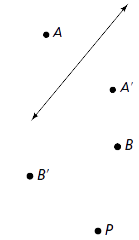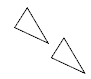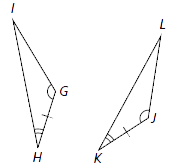Display Accessibility Tools

# 8-5 Butterflies, Pinwheels and Wallpaper - Concepts and Explanations

## Symmetry Transformations

You can use symmetry transformations— reflections, rotations, and translations—to create symmetric designs and to compare the size and shape of figures.

You can specify a reflection by giving the line of reflection.

You can specify a rotation by giving the center of rotation and the angle of the turn.

You can specify a translation by giving the length and direction of the slide. Usually, an arrow with the appropriate length and direction is drawn.

### ExamplePoint A and its reflection image point A� lie on a line that is perpendicular to the line of symmetry and are equidistant from that line.

Point B and its image point B� are equidistant from the center of rotation P. A point under a rotation “travels” on the arc of a circle whose radius is the constant distance between point B and center P.

The set of circles on which the points of a figure “travel” are concentric circles with center P. The angles formed by the vertex points of the figure and their rotation images all have measures equal to the angle of the turn.If you draw the segments connecting a number of points to their images, the segments will be parallel and the same length. The length is equal to the distance of the translation.

## Congruent Figures

Figures of the same size and shape are congruent.

### ExampleYou can “move” one triangle exactly onto the other by a sequence of symmetry transformations.

## Similarity

A two-dimensional figure is similar to another if the second can be obtained from the first by a sequence of rotations, reflections, translations, and dilations.

A dilation enlarges or reduces a figure by a scale factor about a center point so that the original figure and its image are similar. You can specify a dilation by giving the center of dilation and the scale factor.

### ExampleDilations conserve the shape of a figure, but not the size. A scale factor that is greater than 1 stretches the figure. A scale factor that is less than 1 shrinks the figure.